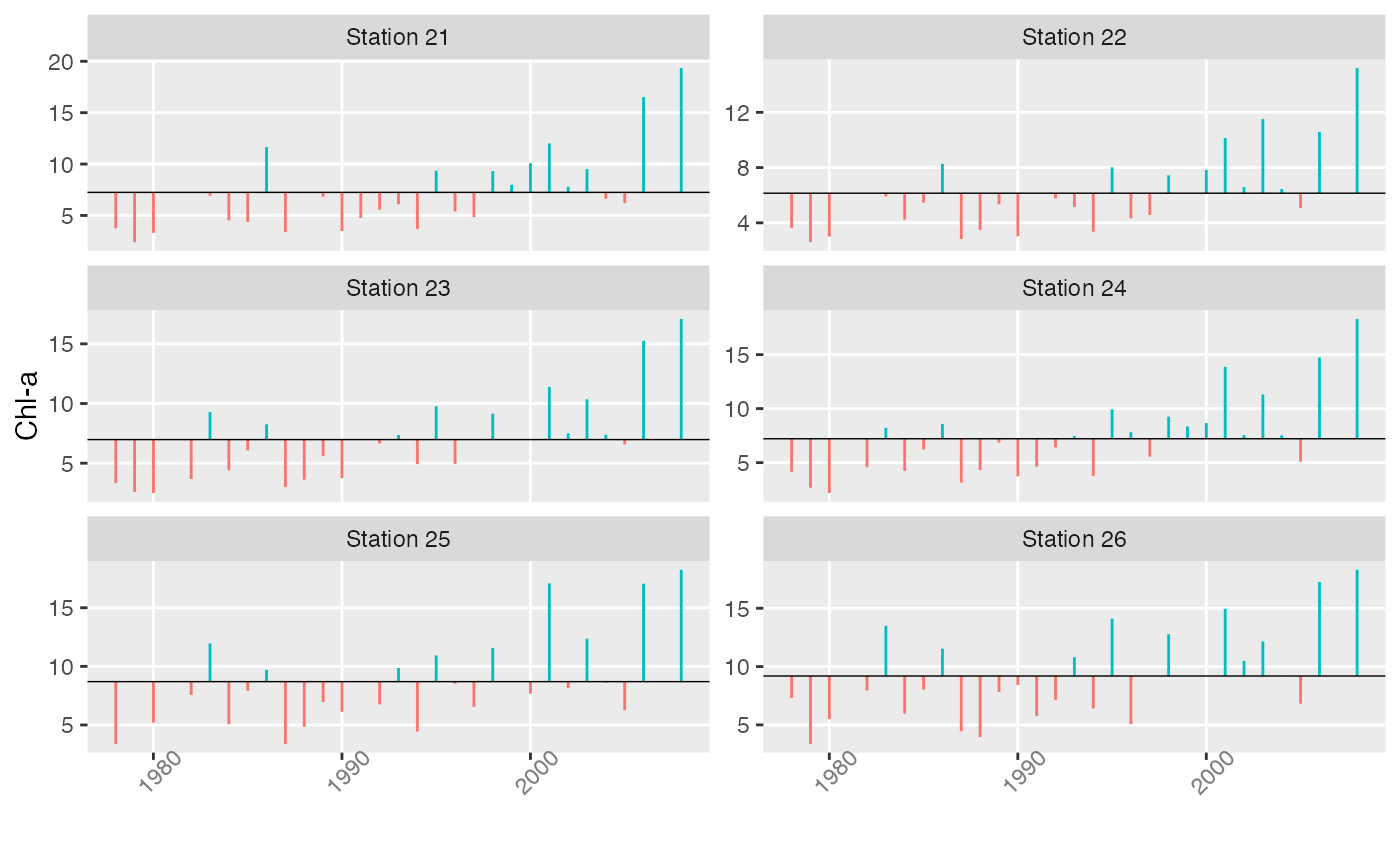Series are illustrated by vertical lines extending from individual data values to the long-term mean. The axes are not scaled in any way. Anomaly plots are useful for visualizing shifts in time series levels.

plotTsAnom(x, xlab = NULL, ylab = NULL, strip.labels = colnames(x), ...)

## Arguments

x matrix or vector time series optional x-axis label optional y-axis label labels for individual time series plots additional options

## Value

A plot and corresponding object of class “ggplot”.

## Details

Options are passed to the underlying facet_wrap function in ggplot2. The main ones of interest are ncol for setting the number of plotting columns and scales = "free_y" for allowing the y scales of the different plots to be independent.

plotTs

## Author

Alan Jassby, James Cloern

## Examples


# Spring bloom size for 6 stations in SF Bay
bloom <- aggregate(sfbayChla[, 1:6], 1, meanSub, sub=3:5)
plotTsAnom(bloom, ylab = 'Chl-a', strip.labels = paste('Station',
substring(colnames(bloom), 2, 3)), ncol = 2, scales = "free_y")